# Run control panel

The run control panel is a notebook containing two tabs. The first of these, headed 'Run control', is the one usually displayed, and provides the most commonly used actions for controlling the execution, as well as indications of progress. The other tab, 'Run settings', gives access to selections which fine-tune the model execution, and will not usually be displayed.

## Run control tab

The run control is used to start and stop the simulation of the model.

• the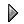play button will begin execution at the current time for a number of time steps set using "Execute for".

• the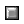stop button will stop the simulation (if one is running) and set the current time to zero.

• the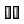pause button will pause the simulation at the current time.

Note that the pause button is only displayed if a simulation is running, and the play button is only displayed if one is not.

The progress bar indicates the elapsed time as a proportion of the total. To the right of the progress bar is an LED which changes colour to indicate the current status of the simulation. This table explains the meanings of the most commonly seen LED colours.

LED colour

Corresponding model statusBlack

Model is stopped and ready to run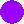Purple

Model is stopped and ready to run, but has been edited since the execution was started so the results might not correspond to the current model diagram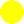Yellow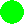Green

Model is executingBlue

Display tools are busy updating their images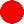Red

The model has been stopped, either by executing the stop(...) function, or by a mathematical error, and needs to be reset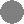Grey

The model code is loaded but some fixed parameter values must be supplied for it to run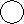White

The model has been stopped by a program error, and the executable code needs to be rebuilt

During a long run, the LED may appear some shade between green and blue; this can be interpreted as indicating what proportion of time Simile is spending actually executing the model as opposed to redrawing the displays of the visualization tools. From version 5.4 onwards, Simile is capable of running the model and updating its displays simultaneously when running on a multi-CPU computer; the LED is blue while doing both at once.

The other fields on this tab are:

• Execute for

This setting controls the number of time units for which the model is run each time you push the "play" button. You can enter a negative number here to use your model for backcasting, i.e., extrapolating the past from the present.

• Current time

This setting displays the current number of elapsed time units. It is possible to enter a value into this field, if you wish to begin a simulation at some time other than zero. Note, however, that unless your model contains events that occur at some pre-defined time, this is unlikely to make any difference.

• Display interval

During the course of a simulation run, each helper extracts values from the model for whatever variables it is displaying. This happens at an interval determined by the value in this field. The display interval is specified as a fraction (or multiple) of the time unit. The display interval cannot be shorter than the shortest time step. Setting a short display interval will cause fast-changing values to be displayed with more detail, but will slow down execution of the model.

Starting with version 6.0, there is a checkbox to the left of the display interval field, labelled 'event;' if this is checked, the state of the display tools will also be updated whenever a discrete event occurs in the model. This is particularly useful in two cases: where values of events are being recorded, since an event only has a value at the exact point in time at which it occurs; and when an event causes a change in direction of a continuously varying component, in which case this option allows a graph point to be added at the exact position of the direction change.

• Time step

The time step for a simulation model is the fraction of the unit of time at which all variables are updated within the model. For example, imagine a model consisting of a single compartment which is initially empty, and a flow into the compartment at a rate of 2 litres per day. In this case, if the unit of time is set to be the day, and a time step of 0.1 days is chosen, then Simile will calculate the change to the compartment every 1/10th of a day. Thus, after the first time step, the container would contain 0.2 litres, i.e. 1/10 of 2 litres, 0.4 litres after the next time step, and so on. After one day (ten time steps) the compartment will contain 2 litres.

## Run settings tab

The following actions are available on the second tab of the run control panel:

• Select time units

This setting is for use with physical units, to specify the particular time unit, rather than using abstract time units. If you have not specified consistent physical units for all flows, you should not change this setting from the default "unit". If you have specified consistent physical units, you should choose the unit you wish to use to specify how long to execute the model for.

• Integration method

The integration method determines how the update of state variables is calculated on each time step. If your model uses Systems Dynamics elements only (i.e. compartments, flows and variables) then you will find Runge-Kutta to be more accurate with less computational effort. For all other models, Euler integration will be more reliable. Please see the page on Runge-Kutta integration for more details.

Some problem domains have behaviour that alters between slow, steady changes and rapid fluctuations. These are known as 'stiff systems' and one way to model them accurately is to use adaptive step size variation. What happens is that the model execution monitor makes an estimate of the integration error after each time step, and if it is greater than a certain limit, it reverts the model to the state it was in at the start of that time step and re-does it as two time steps of half the duration. The error estimation and reduction process is repeated for each of those time steps, but if a very low error value is estimated after the second of two equal steps, the time step returns to its previous longer value before execution continues. The value entered in the Time step field is now the maximum length of a time step.

To enable adaptive step size variation, check the box to the left of the word "Adaptive;" and enter a suitable error limit in the entry box to the right. Note that the error limit is used as an absolute value, so models with large numerical values will tend to be run with shorter time steps than those with small numerical values. This changes with Simile v5.7: the error sensitivity now depends on the difference between the user-supplied minimum and maximum values for the compartment (see Equation Dialogue). The larger this difference, the less sensitive the step size will be to integration errors for a given compartment. If either the minimum or maximum value is not specified, it will treat the difference as 100, which results in the same behaviour as for earlier Simile versions.

• Limiting execution speed

Simple models run very, very fast in Simile. This may not always be desirable, for instance if one is trying to control an ongoing simulation in real time by using a slider, or if trying to stop it in response to some event. So it is possible to limit the frequency at which model time steps are executed. To do this, check the box to the left of the label "Limit updates/sec to:" and enter your maximum update rate to the right.

• Time at reset

Normally, the model time after reset is zero. However, it is sometimes useful to have the model time set differently at reset, for instance if the model's initial state relates to a historical date and it is parameterized with a time series that is indexed by subsequent dates. In this case, the time units may (or may not) be set to Year, and 'time at reset' set to a given year, so the model time corresponds to the calendar time in the domain being simulated. This will also result in the X axis of graphs, etc, being annotated with values that can be read as the calendar time.

• "Pause on:" occurrences

If an occurrence type is selected here, the model will pause whenever that thing happens. The preferences dialogue includes an option that decides whether a dialogue will be displayed when this happens. In any case an entry will be added to the Log tab, describing what happened, and the model can be restarted by hitting the 'Play' button again. The occurrence types are events (which does not include time series events) and compartment under/overruns. If this is selected, the model will pause every time a compartment goes below its minimum or above its maximum value, as set in the equation dialogue. This can be useful for debugging models.

## Log tab

This tab contains a list of descriptions of things that happen while running the model, including events and compartment under/overruns if they are selected (see above), and user-defined pauses. The contents of the log tab are formatted as a table with fields enclosed in curly brackets, and can be cut and pasetd into other applications, e.g., spreadsheets.

## Further notes

### What display interval should I use?

When choosing a display interval, bear in mind the following factors:

• If it is very small, then you will slow down the simulation. Updating the display is relatively time-consuming, and the smaller the display interval, the more frequently this happens.

• If it is too large, you may miss important dynamics, especially in a simulation that shows sudden swings in behaviour. An extreme case of this is when you are simulating switching behaviour: for example, in simulating overflow of a container with fixed capacity by allowing an overflow to operate when the contents of the container exceeds a maximum. In this case, you may get a very misleading graph for the overflow (perhaps showing extended periods of no overflow followed by extended periods of an overflow). If you do obtain apparently aberrant behaviour, then set the display interval to be the same as the time step, so that you are quite sure that you are seeing exactly what is happening to the variable.

Observing the proportion of time that the LED status indicator is blue will give you an idea of how important it is to choose the optimum display interval. If the status indicator is blue most of the time you will be able to improve the performance significantly by increasing the display interval. If it is green most of the time, increasing the display interval will have little effect.

### Time steps

If the processes that you are simulating in your model are truly continuous, then you are actually specifying a model based on differential equations, with compartments corresponding to state variables. In general, digital computers cannot solve such models exactly, so they resort to various numerical methods for calculating the value of the state variable forward through time. There is a large numbers of such methods, with some of them producing far greater accuracy for a given amount of computational effort than others. Simile uses the simplest such method, the Euler method. The reason for this is that, in general, a Simile model can contain features that make it unsuitable for solving through other, more advanced, numerical methods.

### What time step should I use?

There is no simple answer to this question. If the processes you are modelling happen on a discrete-time basis - for example, animals reproducing once per year - then you can use a time step of 1. Otherwise, explore the effect of reducing the time step. If you get no significant difference in model behaviour, then use the bigger time step. If you do get a significant difference, then keep on reducing the time step until you cease to get a change in behaviour.

A useful rule-of-thumb is that no state variable should change by more than two parts in one hundred over one time step.

### Why is the time step labelled as being "Time step #1"?

Different submodels in a model can be specified as operating on different time steps (in the submodel properties dialogue). For example, you might have a model with a human demographic submodel operating on an annual time step, and a lake pollution submodel operating on a 1/100th of a year basis. In that case:

Time step #1 = 1

Time step #2 = 0.01

### How does adaptive step size variation work?

Simile's adaptive step size variation mechanism differs from other implementations. How it works is, at the end of a time step, a compartment's rate of change is calculated and compared with what it was expected to be when its value at that time was calculated. In the simple case of Euler integration, a compartment's value is calculated with the assumption that its rate of change stays the same throughout the time step, so the estimated error is the difference between the new rate of change and the previous one, times the current step size. Error estimation for Runge-Kutta integration works in a way analogous to this, but the estimated rate of change is a function of the intermediate rates of change generated during the application of that method.

## Back-casting

Entering a negative number in the "Execute for..." field in the Run Control enables certain back-casting calculations to be performed. Time will obey the usual rule of stepping from the "Current time" (which you can enter) in steps of magnitude given by "Time step" for the total number of units specified by "Execute for". For example, you could request execution for 100 time units, enter a current time of -100, and a time step of 0.1. Simile will then perform as it usually does by default with 1000 calculations, but in reverse.

In the model equations, you have entered "Initial values" for the compartments (state variables). When the model is first built, or subsequently reset, the "Current time" is set to zero and the compartments are allocated these initial values. Even if the "Current time" is manually changed after this, the compartment values are not changed. It is therefore not possible to enter time-dependent initial values in the model. On the other hand, if you want to enter "Initial values" that represent the state of the system at some non-zero time, this can be achieved by altering the "Current time" setting after the model is built or reset.

If, however, the model is not reset, then the state variables will retain their values from the end of the previous run. This allows a model's calculations to be repeated in reverse, simply by reversing the sign of the time step. (This is a good check on the numerical accuracy of the solution: if the initial values are not re-calculated exactly at the end of the reverse calculation, then the difference is equal to double the error.)

Note: Only models consisting of System Dynamics elements only can reasonably be treated in this way. If population submodels are used, it is not possible to reverse the action of the population control elements through time.

This works with both Euler and Runge-Kutta integration, and given the restriction above, it can usually be assumed that Runge-Kutta integration is preferable.

# Runge-Kutta integration

Runge-Kutta integration provides more accurate values for a given level of computational effort than Euler integration, for those models involving only systems of differential equations. Simile always uses Euler integration by default, because it is applicable to any model.

For example, consider the equation dy/dt = t, and given y = 0 at t = 0. In this case, there is an analytical solution, y = ½t2, and therefore at t = 100, y = 5000. Using the Euler integration algorithm, and using a step-size of 0.1 (the default) Simile calculates the answer to be 4995. Using Runge-Kutta integration, the correct answer of 5000 is found using the same step size (and therefore approximately the same computational effort). Even using a step size of 0.01 the answer is calculated to be 4999.5, using Euler integration.

However, it must be noted that Runge-Kutta integration is not always applicable, unlike Euler integration, which can be used to solve any system of differential or difference equations.

In particular, Simile allows the construction of difference equations, where certain quantities accumulate in sudden increments corresponding to weeks, months or years, for example. Interest payments, in particular, correspond to this method of accumulation. These systems must be solved by Euler integration, with the step size chosen to correspond to the periodicity.

Furthermore, although Runge-Kutta integration does not fail when used with discontinuous functions (such as are involved in the creation and destruction of instances of population submodels) the results will be no more precise than using Euler integration.

The choice between Euler and Runge-Kutta integration is made at run time, using a drop-down box in the Run Control. The chosen method is saved with the model and restored when the model is loaded. The user can experiment with running a model using either method, without having to rebuild the model, to understand whether a significant improvement is achieved.